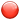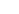## Training Snap Shot: BODMAS and BIDMAS

### Why is BODMAS or BIDMAS is so important?

Because it lets you know the order to solve a mathematical sum. It's important that you follow the order of BODMAS/BIDMAS or your answer will be wrong.

#### BODMAS/BIDMAS stand for:

•Brackets
•Order/Indices (Power)
•Divide
•Multiplication
•Addition
•Subtraction

• Let’s look at these examples:

•Not using BODMAS:
2 + 2 x 2 = 8 WRONG!!
•Using BODMAS
2 + 2 x 2 = 6 remember Multiplication before Addition
•Using BODMAS with a sum with brackets:
(2 + 2) x 2 = 8
Brackets first (2 + 2) = 4, then 4 x 2 = 8 remember Brackets before Multiplication
•You need to follow it in this order Brackets first, Orders ie Powers come second, Division then Multiplication, Addition & then Subtraction is last that is the order in which you need to breakdown & calculate the sum.
•Excel Help File
BODMAS is the order of how formulas work in Excel and Maths in general. It lets you know which order to solve complex sums. When you are solving a formula in Excel it uses BODMAS so you get the result you’re looking for, BODMAS stands for:

Always remember to start the formula off with the equals sign.

It can also be known as BIDMAS, in this case the Oder becomes Indices, they have the same meaning but some people may know it as the different names.